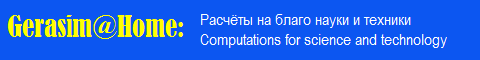Российские распределенные вычисления на платформе BOINCBerkeley Open Infrastructure for Network Computing

 Welcome Guest

Project News

 Tuesday, October 20, 2020 evatutin #98

By analogy with inequalities for loops (see https://vk.com/wall162891802_1403), we can formulate a number of inequalities for Latin subrectangles in DLS. Every nontrivial subrectangle is a subrectangle in the DLS by definition. Similarly, by definition, each intercalate is a nontrivial 2x2 subrectangle (except for the dimension N = 2, where it will be trivial, but there is no DLS of this dimension). These simple statements allow us to establish a number of relationships between the values ​​of numerical series associated with subrectangles and intercalates:

1. For subrectangles: 0 <= A307839(N) <= A307840(N).
2. For nontrivial subrectangles: 0 <= A307841(N) <= A307842(N).
3. For minimum values: 0 <= A307163(N) <= A307841(N) <= A307839(N).
4. For maximum values: A307164(N) <= A307842(N) <= A307840(N).